## Biomedical Research

All submissions of the EM system will be redirected to Online Manuscript Submission System. Authors are requested to submit articles directly to Online Manuscript Submission System of respective journal.

Research Article - Biomedical Research (2018) Artificial Intelligent Techniques for Bio Medical Signal Processing: Edition-II

## Three-dimensional spatial orientation non-visible area for biomedical imaging based on received signal strength indicator

Hejun Zhu and Liehuang Zhu*

School of Computer Science, Beijing Institute of Technology, Beijing, PR China

*Corresponding Author:
Liehuang Zhu
School of Computer Science
Beijing Institute of Technology, PR China

Accepted on August 30, 2017

Visit for more related articles at Biomedical Research

### Abstract

Wi-Fi Direct technology will gradually apply to all kinds of handheld terminals. This paper researches Wi-Fi Direct three-dimensional spatial positioning algorithm on 3D biomedical imaging, combined with a reference node to estimate the distance of unknown nodes, the nodes of the unknown relative positioning. This paper simulates distance correction of both log-distance path loss model and wall attenuation factor model. By RSSI distance measurement model analysis and consequential amendments. The RSSI obtained by at least three known Wi-Fi Direct device from the same unknown Wi-Fi Direct device of a non-visible area are estimated the distance, to achieve three-dimensional spatial positioning of the biomedical imaging device combined with the geometry. Distance measurement model and its amendments are simulated in Wi-Fi Direct architecture based on RSSI and which used to achieve spatial positioning of an unknown device of the non-visible area on biomedical imaging.

## Introduction

As a new kind of technology for short rang wireless communication, Wi-Fi Direct uses a technology of point to point, meaning that Wi-Fi devices without going through a wireless network base station. Wi-Fi Direct does not require access points, so you can connect anywhere. Wi-Fi Direct positioning is based on received signal strength indication to achieve spatial positioning technology, compared with GPS positioning technology, although the positioning accuracy is generally, but Wi-Fi Direct positioning with low cost, ease of use, etc., which can be applied in many medical imaging techniques radionuclide imaging, nuclear imaging system etc. .

This paper researches Wi-Fi Direct three-dimensional spatial positioning algorithm on 3D biomedical imaging, combined with a reference node to estimate the distance of unknown nodes, the nodes of the unknown relative positioning . By RSSI distance measurement model analysis and consequential amendments. The RSSI obtained by at least three known Wi-Fi Direct device from the same unknown Wi-Fi Direct device of a non-visible area are estimated the distance, to achieve threedimensional spatial positioning of the biomedical imaging device combined with the geometry [3,4]. Use of RSSI distance measurement model can obtain good spatial resolution in nuclear imaging techniques. Fast geometry acquisition in medical imaging devices enables the early detection, more reliability in motion tracking etc. [5,6].

## Methods

The distance measurement based on the received signal strength indicator is made the signal strength indicator between the transmitter and receiver indicated by the corresponding model into the transmission distance, and then estimates the location of the node. However, in practical medical imaging applications, different environmental factors affect the wireless signal in the transmission, different environmental factors, and single measurement range model will be different for texture difference from the same environment leading to the attenuation of the signal is different, and therefore the distance estimation based on received signal strength indication is a big error.

In order to make the model not only can reflect the characteristics of the indoor environment, at the same time, it can ensure that the RSSI distance measurement is more accurate, both A value and n value need to refine continuously based on the specific environment, in order to make the A value and n value is more suitable for the indoor environment parameters. A value and N value can be estimated by linear regression analysis, n is the signal strength of the sample number. Then there are the following formulas (1) and (2) for estimating: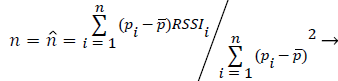(1)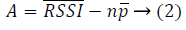(3)(4)

True coefficient indicates the regression coefficient, defined as formula (5).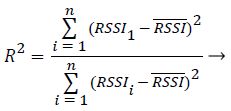(5)

Wherein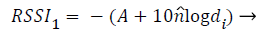(6)

Standard deviation of predictive value of received signal strength indicator is as formula (7),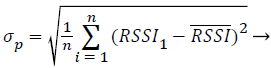(7)

We measured 100 sets of data in the corridor, the value of A is 41 by the formula (2), and the value of n is 2.3 by the formula (1). The most common and effective method to decrease the error is to measure the multigroup data. Depending on the formula (8), calculate all of the received signal strength indicator value for the average value, where xi represents the i received signal strength indicator value.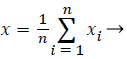(8)

Expression 8 can be seen that the measurement results are closely related to the n, the greater the value of n, the higher the accuracy of the data.

## Results

Simulation of mending for biomedical imaging distance measurement based on RSSI

We may combine Formula (4) and (14) to obtain more accurate path loss exponent n, is able to come to a more accurate the logarithmic distance path loss model, thereby improving the accuracy of distance measurement. For example, about 30m away from the transmitting equipment at the open area in front of the obstacle the path loss exponent n=1.9, as amended by the actual detected and mended after an obstacle the path loss exponent n=3.3. The path loss model before and after the obstacle shown in Figure 1.

Figure 1: Parameter correction based on the logarithmic distance path loss model before and after obstacle.

Simulation of three-dimensional spatial orientation

Three known reference point is added in the group by an unknown point (Wi-Fi Direct device) built, three known reference points according to the corresponding distance measurement model and its amendments will convert the RSSIs from the same unknown point to distances. Such as the known reference point A converts the RSSI to the distance d1, so the unknown point should be on the sphere, the reference point A is the center and d1 si the radius of the sphere. Thus, the three disparate reference points constructs three spheres according to the distances. Every reference point have threedimensional spatial location algorithm, outline the spatial structure according to the corresponding distance data, and then Combined predicted direction of the unknown point can determine (show) the spatial position of the unknown reference point the relative to the three known reference points, as shown in Figure 2.

Figure 2: Space schematic diagram built by RSSIs of three known reference points received form the same unknown point.

## Conclusion

As a new kind of technology for short rang wireless communication, Wi-Fi Direct uses a technology of point to point, meaning that Wi-Fi devices without going through a wireless network base station. Wi-Fi Direct does not require access points, so you can connect anywhere. Wi-Fi Direct positioning is based on received signal strength indication to achieve spatial positioning technology, compared with GPS positioning technology, although the positioning accuracy is generally, but Wi-Fi Direct positioning with low cost, ease of use, etc., which can be applied in many medical imaging applications.